``````library("ggplot2")
library("microbenchmark")
# knitr::opts_chunk\$set(eval = FALSE)

autoplot.microbenchmark <- function(obj) {
levels(obj\$expr) <- paste0("Expr_", seq_along(levels(obj\$expr)))
microbenchmark:::autoplot.microbenchmark(obj)
}

speed_up <- function(obj) {
levels(obj\$expr) <- paste0("Expr_", seq_along(levels(obj\$expr)))
obj <- as.data.frame(obj)
summaries <- do.call(rbind, by(obj\$time, obj\$expr, summary))
res <- c()
for (i in seq_len(nrow(summaries)-1)+1) {
res <- rbind(res, summaries[1,] / summaries[i,])
}
rownames(res) <- levels(obj\$expr)[-1]
return(res)
}

restore_sess <- function() {
return()
env <- .GlobalEnv
rm(list=setdiff(ls(envir = env), c(
"autoplot.microbenchmark",
"speed_up",
"n",
"restore_sess"
)),
envir = env
)
}
n <- 1000``````

## Specific techniques

### Loop optimizations

#### Induction variable analysis

``# Roughly, if a variable in a loop is a simple linear function of the index variable, such as `j := 4*i + 1`, it can be updated appropriately each time the loop variable is changed. This is a strength reduction, and also may allow the index variable's definitions to become dead code. This information is also useful for bounds-checking elimination and dependence analysis, among other things.``
``````# 1
restore_sess()
mb_res <- microbenchmark(
{
j = -17;
for (i in 0:9) {
j = j + 17;
}
},{
j = -17;
i <- 0:9
j <- j + 17*length(i)
}
)
autoplot(mb_res)``````
``## Coordinate system already present. Adding new coordinate system, which will replace the existing one.``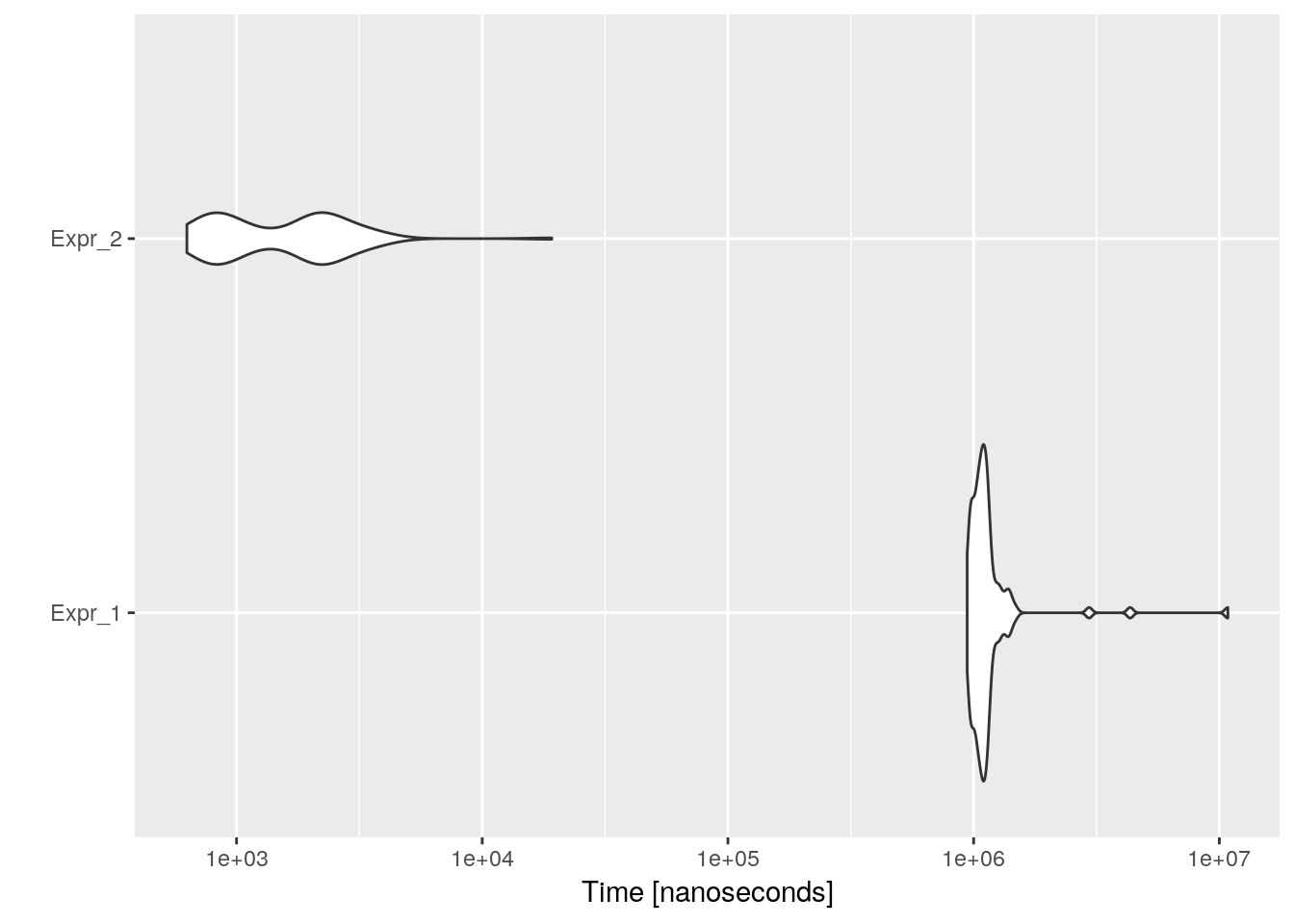``speed_up(mb_res)``
``````##            Min.  1st Qu.   Median     Mean  3rd Qu.     Max.
## Expr_2 1498.588 1216.418 581.7741 666.6875 494.7002 564.7007``````

#### Loop fission or loop distribution

``# Loop fission attempts to break a loop into multiple loops over the same index range but each taking only a part of the loop's body. This can improve locality of reference, both of the data being accessed in the loop and the code in the loop's body.``
``````# 1
restore_sess()
mb_res <- microbenchmark(
{
a <- c()
b <- c()
for (i in 1:100) {
a[i] = 1;
b[i] = 2;
}
},{
a <- c()
b <- c()
for (i in 1:100) {
a[i] = 1;
}
for (i in 1:100) {
b[i] = 2;
}
}
)
autoplot(mb_res)``````
``## Coordinate system already present. Adding new coordinate system, which will replace the existing one.``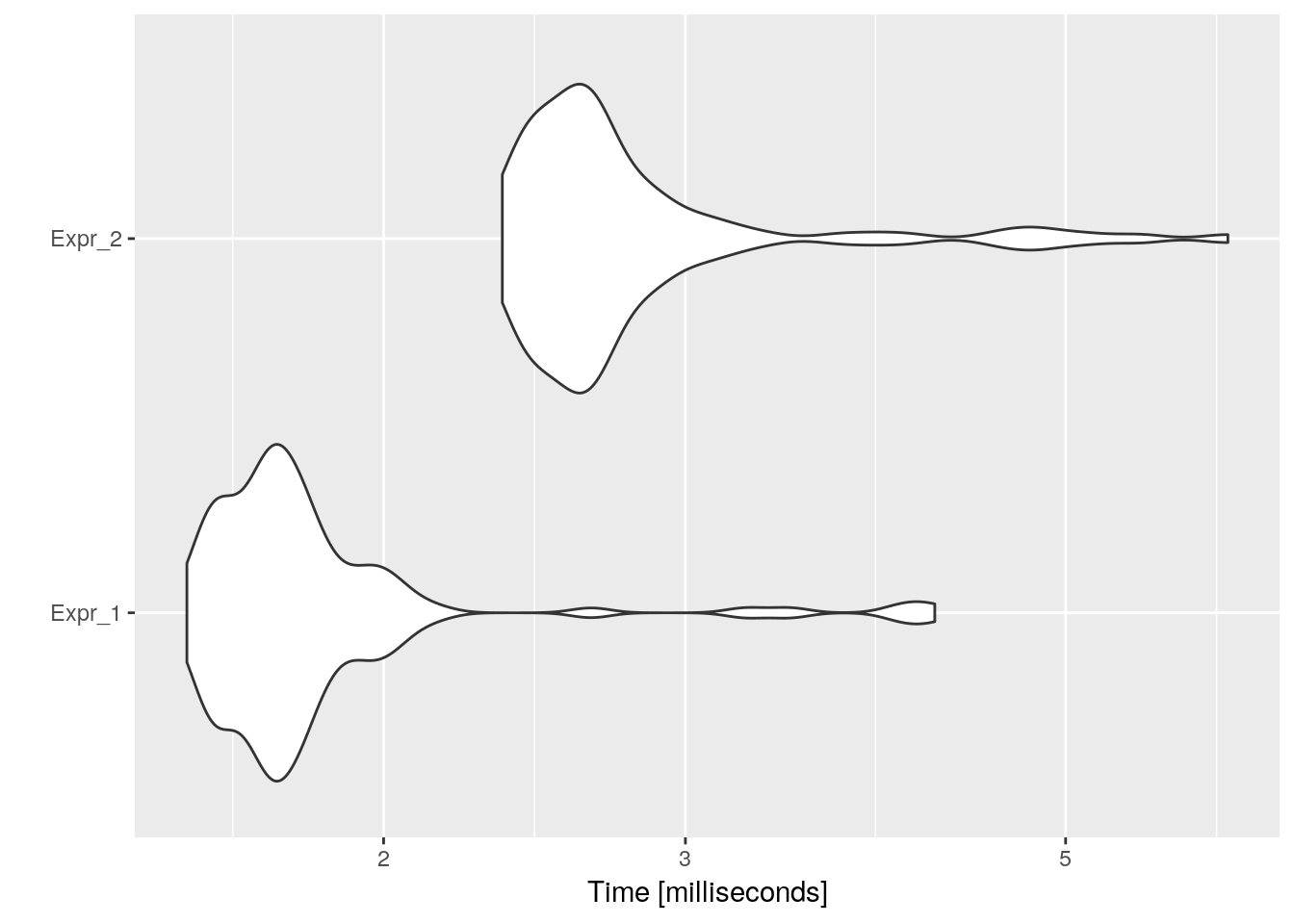``speed_up(mb_res)``
``````##             Min.   1st Qu.    Median      Mean   3rd Qu.      Max.
## Expr_2 0.6545591 0.6673629 0.6606564 0.6528722 0.6527903 0.6744943``````

#### Loop fusion or loop combining or loop ramming or loop jamming

``# Another technique which attempts to reduce loop overhead. When two adjacent loops would iterate the same number of times (whether or not that number is known at compile time), their bodies can be combined as long as they make no reference to each other's data.``
``````# 1
restore_sess()
mb_res <- microbenchmark(
{
a <- c()
b <- c()
for (i in 1:100) {
a[i] = 1;
}
for (i in 1:100) {
b[i] = 2;
}
},{
a <- c()
b <- c()
for (i in 1:100) {
a[i] = 1;
b[i] = 2;
}
}
)
autoplot(mb_res)``````
``## Coordinate system already present. Adding new coordinate system, which will replace the existing one.``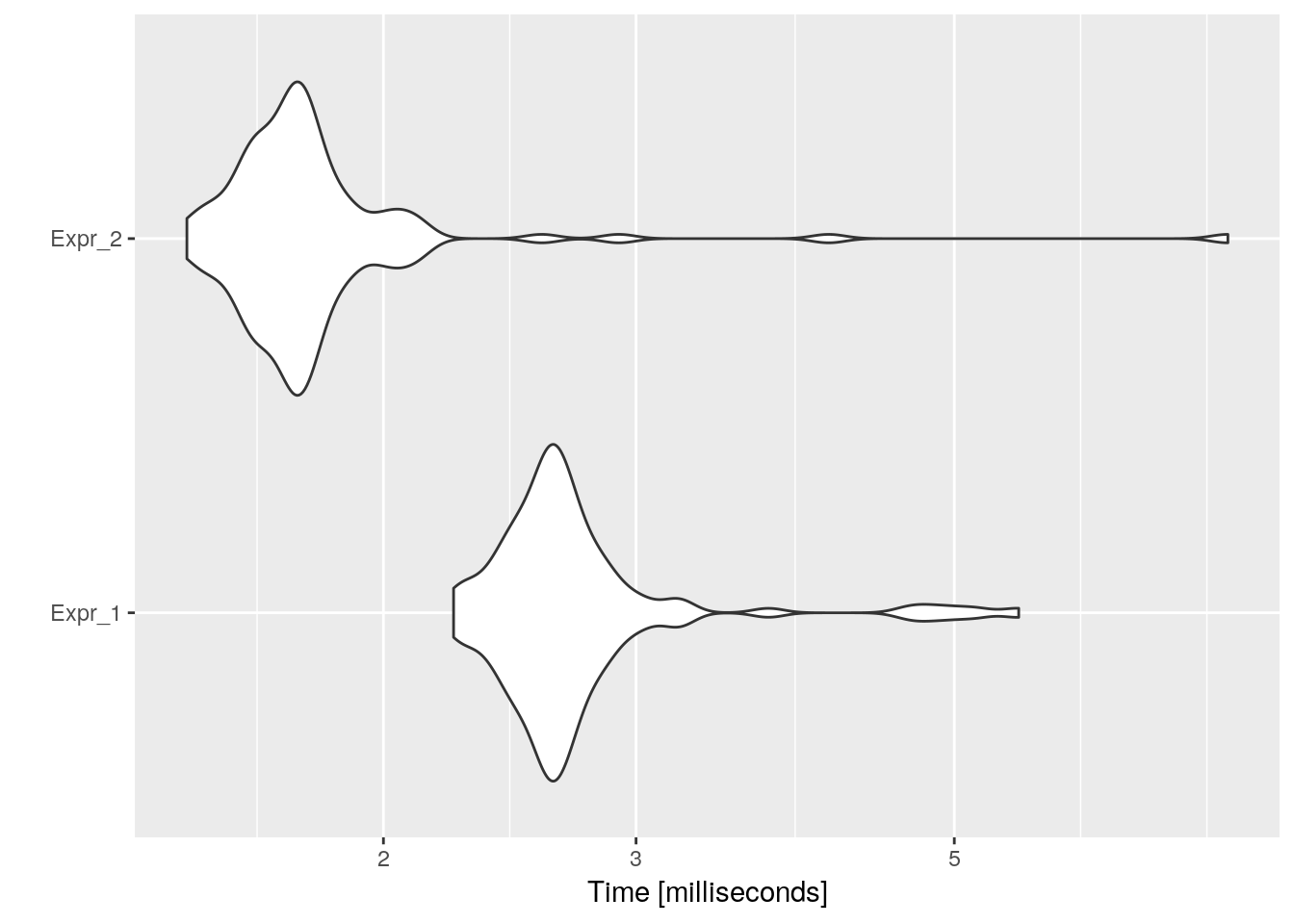``speed_up(mb_res)``
``````##            Min.  1st Qu.   Median     Mean  3rd Qu.     Max.
## Expr_2 1.534242 1.532572 1.514205 1.498098 1.525176 0.714802``````

#### Loop inversion

``# This technique changes a standard while loop into a do/while (also known as repeat/until) loop wrapped in an if conditional, reducing the number of jumps by two, for cases when the loop is executed. Doing so duplicates the condition check (increasing the size of the code) but is more efficient because jumps usually cause a pipeline stall. Additionally, if the initial condition is known at compile-time and is known to be side-effect-free, the if guard can be skipped.``

Comment: NA

#### Loop interchange

``# These optimizations exchange inner loops with outer loops. When the loop variables index into an array, such a transformation can improve locality of reference, depending on the array's layout.``
``````# 1
restore_sess()
mb_res <- microbenchmark(
{
a <- matrix(nrow = 10, ncol = 20)
for (i in 0:10) {
for (j in 0:20) {
a[i, j] <- i+j
}
}
},{
a <- matrix(nrow = 10, ncol = 20)
for (j in 0:20) {
for (i in 0:10) {
a[i, j] <- i+j
}
}
}
)
autoplot(mb_res)``````
``## Coordinate system already present. Adding new coordinate system, which will replace the existing one.``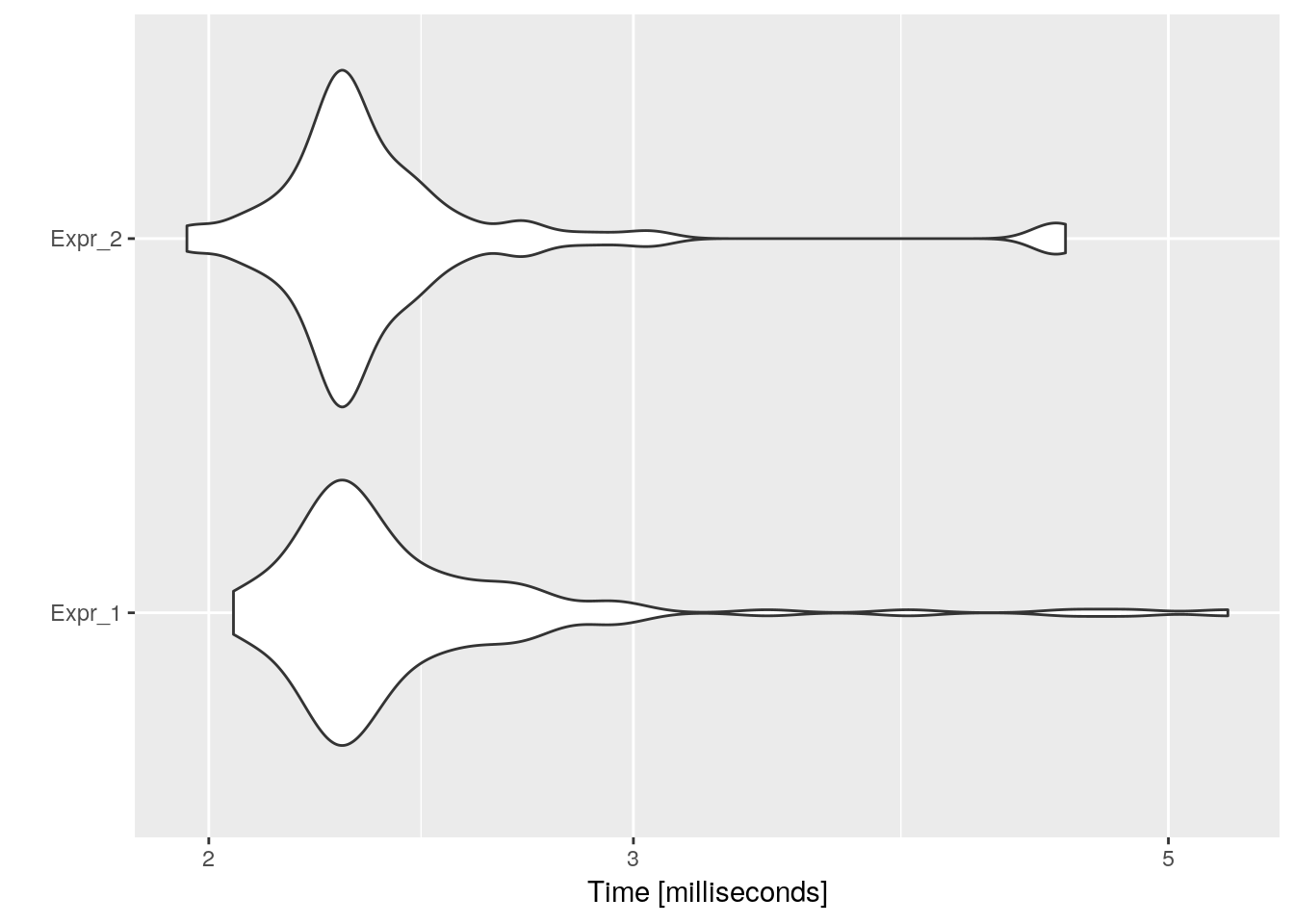``speed_up(mb_res)``
``````##            Min.  1st Qu.  Median     Mean  3rd Qu.     Max.
## Expr_2 1.045462 1.002313 1.00738 1.020242 1.032166 1.167716``````

#### Loop-invariant code motion

``# If a quantity is computed inside a loop during every iteration, and its value is the same for each iteration, it can vastly improve efficiency to hoist it outside the loop and compute its value just once before the loop begins. This is particularly important with the address-calculation expressions generated by loops over arrays. For correct implementation, this technique must be used with loop inversion, because not all code is safe to be hoisted outside the loop.``
``````# 1
restore_sess()
y <- rnorm(1)
z <- rnorm(1)
mb_res <- microbenchmark(
{
i = 0;
a <- c()
while (i < n) {
x = y + z;
a[i] = 6 * i + x * x;
i <- i+1;
}
},{
i = 0;
a <- c()
x = y + z; # be careful, if i > n then x doesnt have to exist!!
while (i < n) {
a[i] = 6 * i + x * x; # we could define aux <- x*x outside the loop
i <- i+1;
}
},{
i = 0;
a <- c()
x = y + z; # be careful, if i > n then x doesnt have to exist!!
aux <- x * x
while (i < n) {
a[i] = 6 * i + aux;
i <- i+1;
}
rm(aux)
}
)
autoplot(mb_res)``````
``## Coordinate system already present. Adding new coordinate system, which will replace the existing one.``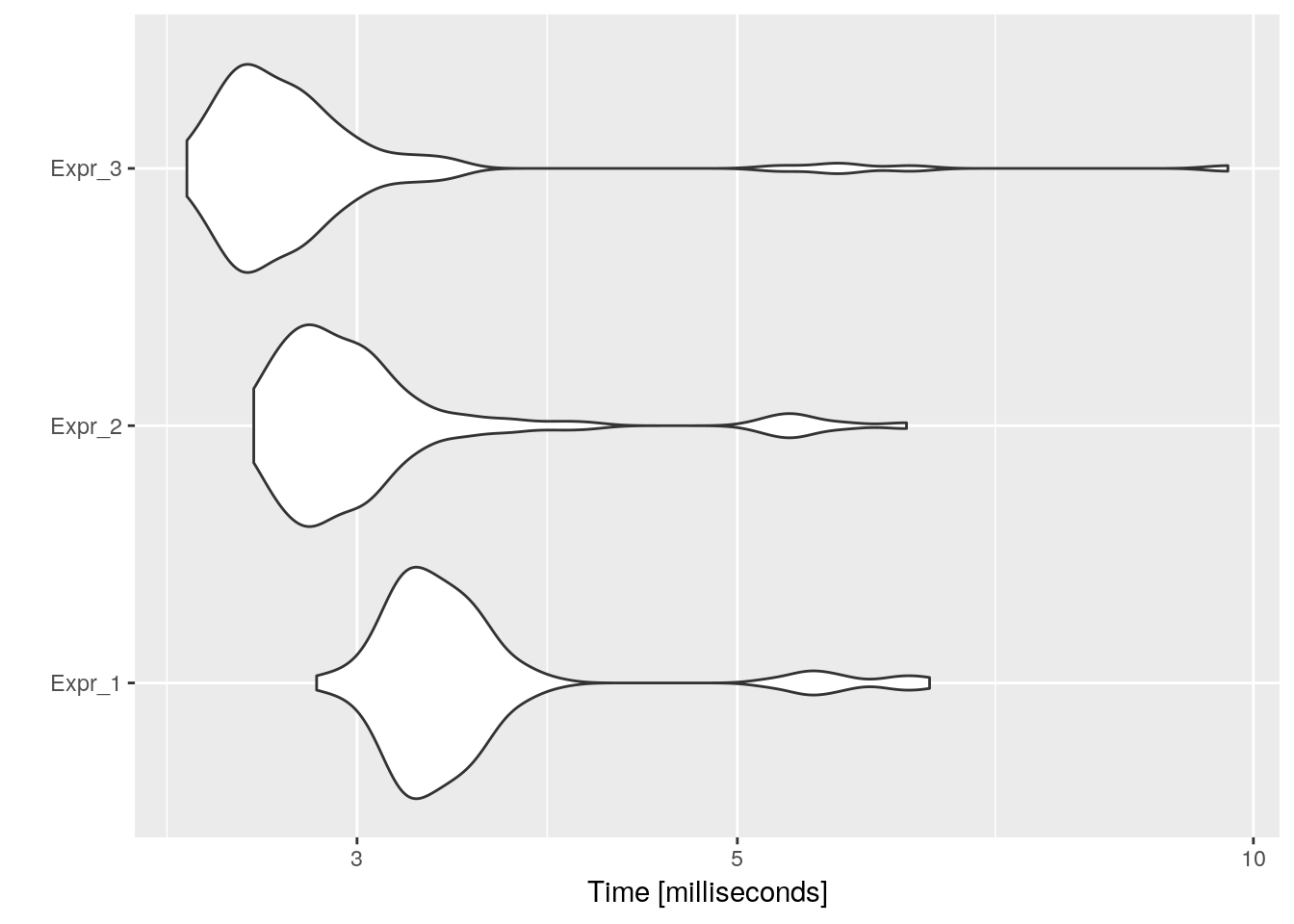``speed_up(mb_res)``
``````##            Min.  1st Qu.   Median     Mean  3rd Qu.      Max.
## Expr_2 1.088090 1.145896 1.141391 1.131066 1.135350 1.0314901
## Expr_3 1.190335 1.246563 1.247391 1.218663 1.231293 0.6698721``````

#### Loop nest optimization

``# Some pervasive algorithms such as matrix multiplication have very poor cache behavior and excessive memory accesses. Loop nest optimization increases the number of cache hits by performing the operation over small blocks and by using a loop interchange.``
``````# 1
restore_sess()
n1 <- 100
a <- matrix(rnorm(n1*n1), ncol = n1, nrow = n1)
b <- rnorm(n1)
c <- numeric(n1)
mb_res <- microbenchmark(
{
for (i in seq(1, n1)) {
c[i] = 0;
for (j in seq(1, n1)) {
c[i] = c[i] + a[i,j] * b[j];
}
}
},{
for (i in seq(1, n1, by = 2)) {
c[i] = 0;
c[i + 1] = 0;
for (j in seq(1, n1, by = 2)) {
for (x in seq(i, min(i + 2, n1))) {
for (y in seq(j, min(j + 2, n1))) {
c[x] = c[x] + a[x,y] * b[y];
}
}
}
}
}
) # not getting the same results, but not faster anyways
autoplot(mb_res)``````
``## Coordinate system already present. Adding new coordinate system, which will replace the existing one.``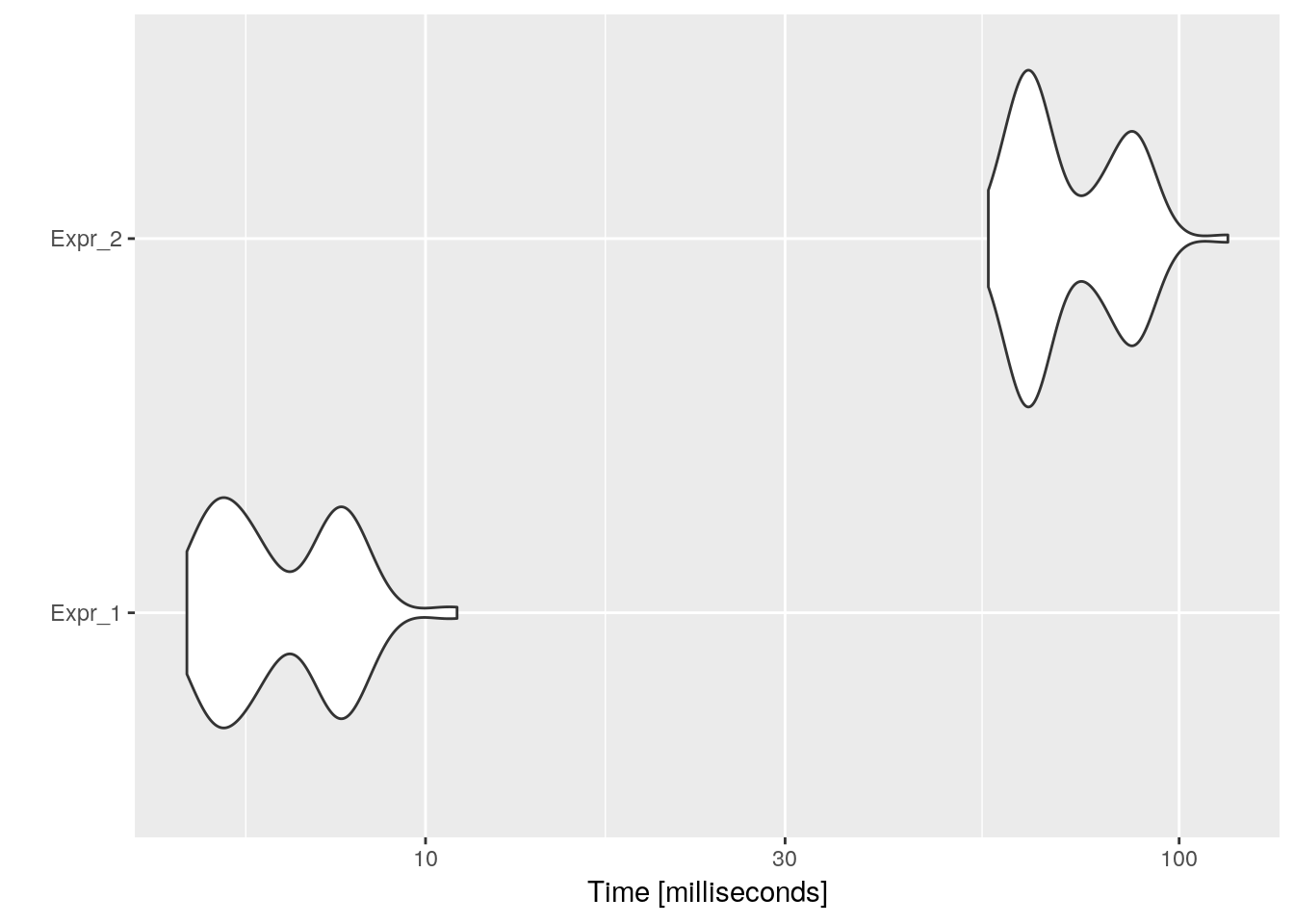``speed_up(mb_res)``
``````##              Min.    1st Qu.     Median       Mean    3rd Qu.       Max.
## Expr_2 0.08639623 0.08601254 0.09161603 0.09016031 0.08932663 0.09486677``````

#### Loop reversal

``# Loop reversal reverses the order in which values are assigned to the index variable. This is a subtle optimization which can help eliminate dependencies and thus enable other optimizations. Furthermore, on some architectures, loop reversal contributes to smaller code, as when the loop index is being decremented, the condition that needs to be met in order for the running program to exit the loop is a comparison with zero. This is often a special, parameter-less instruction, unlike a comparison with a number, which needs the number to compare to. Therefore, the amount of bytes needed to store the parameter is saved by using the loop reversal. Additionally, if the comparison number exceeds the size of word of the platform, in standard loop order, multiple instructions would need to be executed in order to evaluate the comparison, which is not the case with loop reversal.``

#### Loop unrolling

``# Unrolling duplicates the body of the loop multiple times, in order to decrease the number of times the loop condition is tested and the number of jumps, which hurt performance by impairing the instruction pipeline. A "fewer jumps" optimization. Completely unrolling a loop eliminates all overhead, but requires that the number of iterations be known at compile time.``
``````# 1
restore_sess()
mb_res <- microbenchmark(
{
aux <- rnorm(100)
for (x in seq(1, 100)) {
aux[x] <- 0
}
},{
aux <- rnorm(100)
for (x in seq(1, 100, by = 5)) {
aux[x] <- 0
aux[x+1] <- 0
aux[x+2] <- 0
aux[x+3] <- 0
aux[x+4] <- 0
}
}
)
autoplot(mb_res)``````
``## Coordinate system already present. Adding new coordinate system, which will replace the existing one.``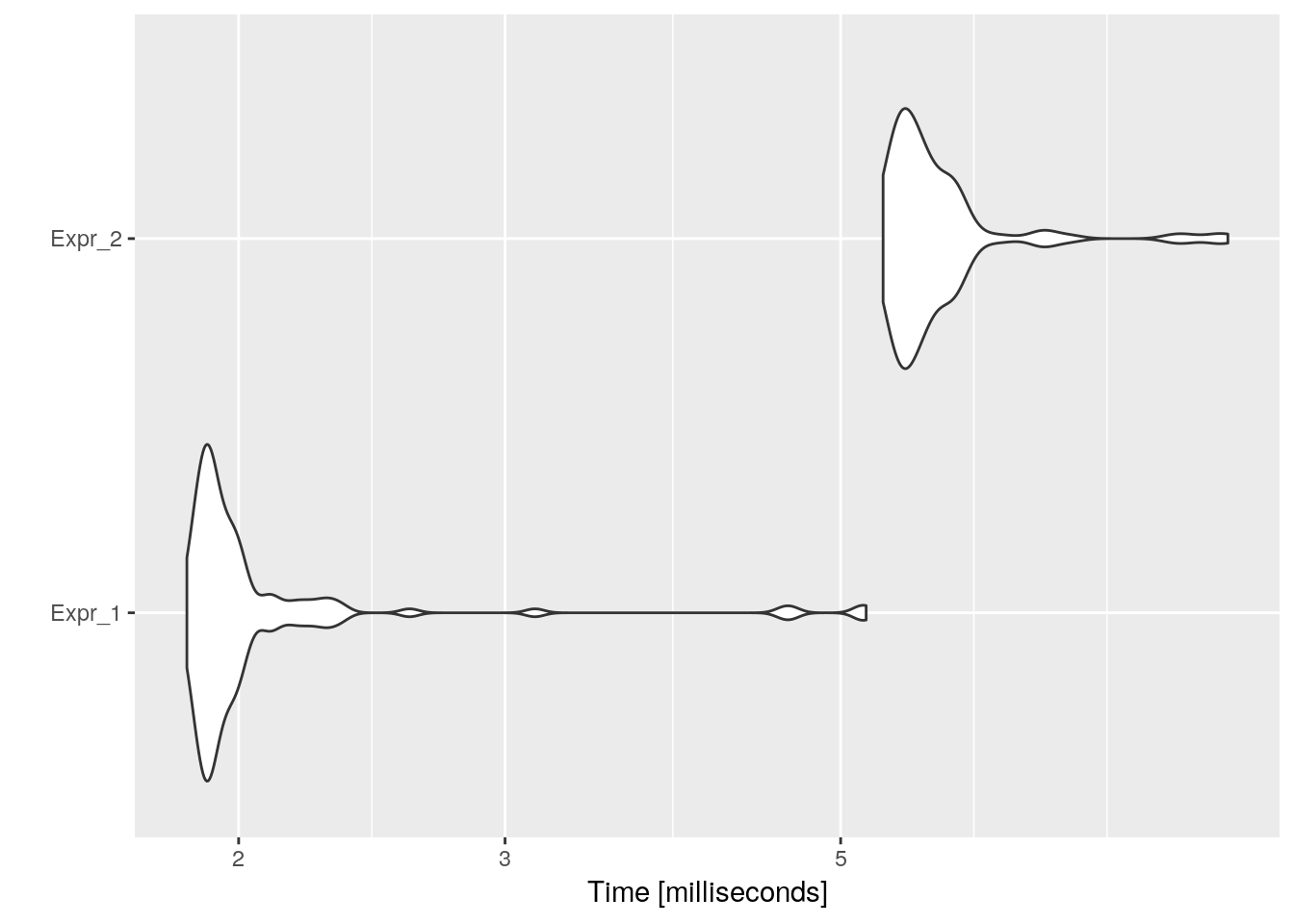``speed_up(mb_res)``
``````##             Min.   1st Qu.    Median      Mean   3rd Qu.      Max.
## Expr_2 0.3465793 0.3464006 0.3440402 0.3618334 0.3389907 0.5766175``````

#### Loop splitting

``# Loop splitting attempts to simplify a loop or eliminate dependencies by breaking it into multiple loops which have the same bodies but iterate over different contiguous portions of the index range. A useful special case is loop peeling, which can simplify a loop with a problematic first iteration by performing that iteration separately before entering the loop.``
``````# 1
restore_sess()
x <- rnorm(n)
mb_res <- microbenchmark(
{
p = n;
y <- numeric(n)
for (i in 1:n) {
y[i] = x[i] + x[p];
p = i; # add i to variable p
}
},{
y <- numeric(n)
y = x + x[n];
for (i in 2:n) {
y[i] = x[i] + x[i-1];
}
}
)
autoplot(mb_res)``````
``## Coordinate system already present. Adding new coordinate system, which will replace the existing one.``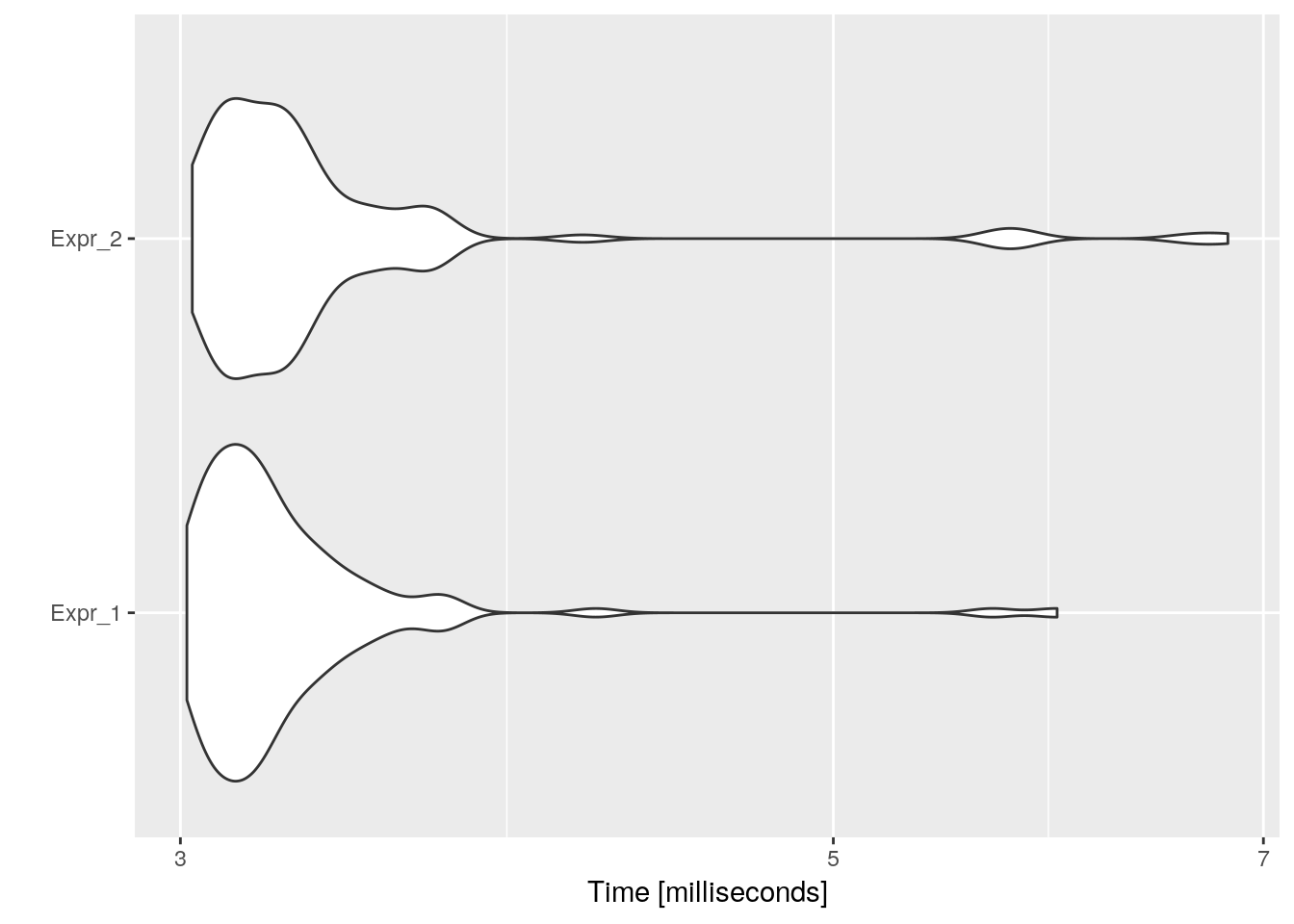``speed_up(mb_res)``
``````##             Min.   1st Qu.    Median      Mean   3rd Qu.      Max.
## Expr_2 0.9958042 0.9947598 0.9809692 0.9627537 0.9848684 0.8749543``````

#### Loop unswitching

``# Unswitching moves a conditional from inside a loop to outside the loop by duplicating the loop's body inside each of the if and else clauses of the conditional.``
``````# 1
restore_sess()
w <- FALSE
mb_res <- microbenchmark(
{
x <- numeric(1000)
y <-  rnorm(1000)
for (i in 1:1000) {
x[i] = x[i] + y[i];
if (w)
y[i] = 0;
}
},{
x <- numeric(1000)
y <-  rnorm(1000)
if (w) {
for (i in 1:1000) {
x[i] = x[i] + y[i];
y[i] = 0;
}
} else {
for (i in 1:1000) {
x[i] = x[i] + y[i];
}
}
}
)
autoplot(mb_res)``````
``## Coordinate system already present. Adding new coordinate system, which will replace the existing one.``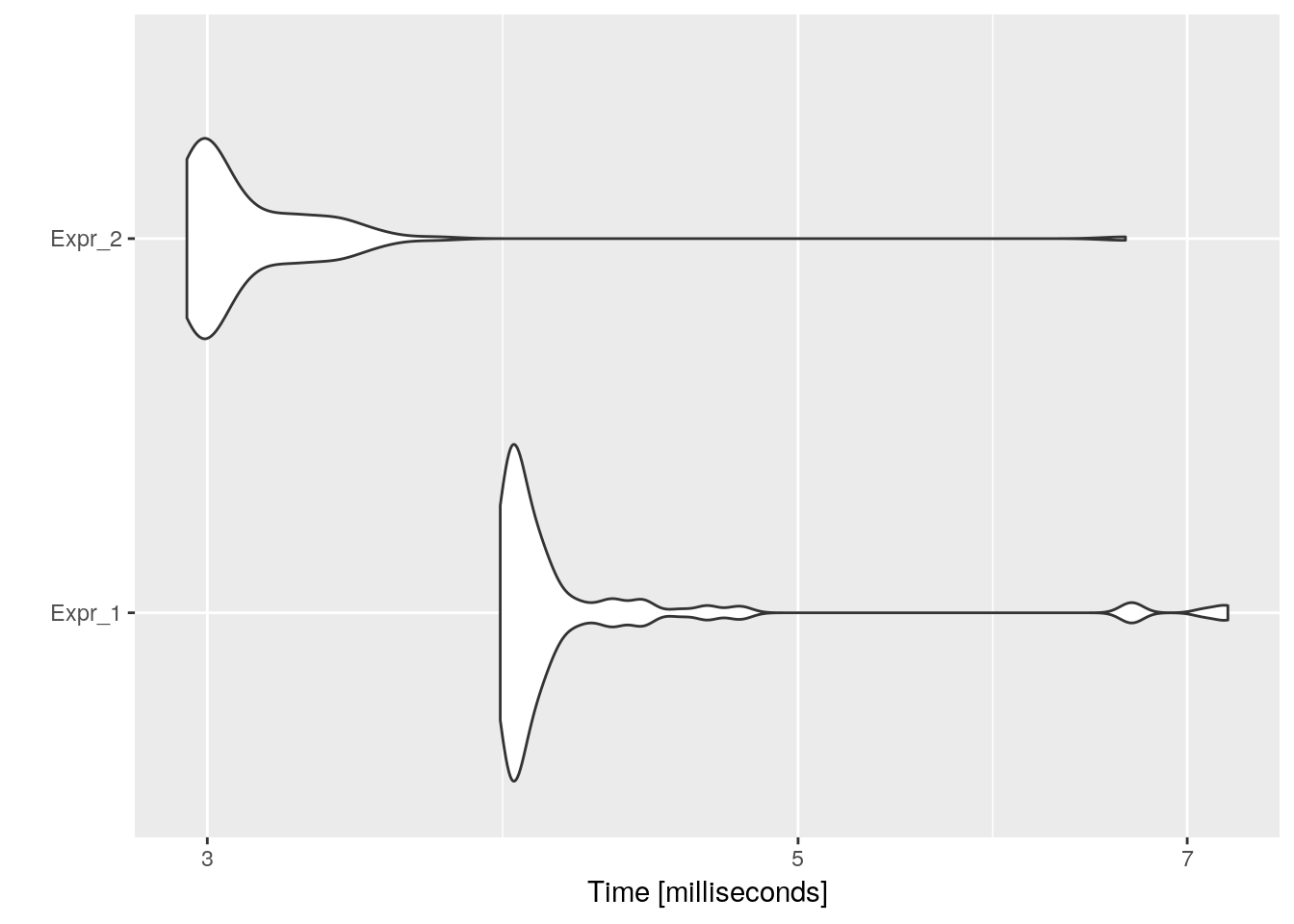``speed_up(mb_res)``
``````##            Min.  1st Qu.   Median     Mean  3rd Qu.    Max.
## Expr_2 1.311213 1.310106 1.304872 1.339897 1.261362 1.09259``````
``````# 2
restore_sess()
w <- !FALSE
mb_res <- microbenchmark(
{
x <- numeric(1000)
y <-  rnorm(1000)
for (i in 1:1000) {
x[i] = x[i] + y[i];
if (w)
y[i] = 0;
}
},{
x <- numeric(1000)
y <-  rnorm(1000)
if (w) {
for (i in 1:1000) {
x[i] = x[i] + y[i];
y[i] = 0;
}
} else {
for (i in 1:1000) {
x[i] = x[i] + y[i];
}
}
}
)
autoplot(mb_res)``````
``## Coordinate system already present. Adding new coordinate system, which will replace the existing one.``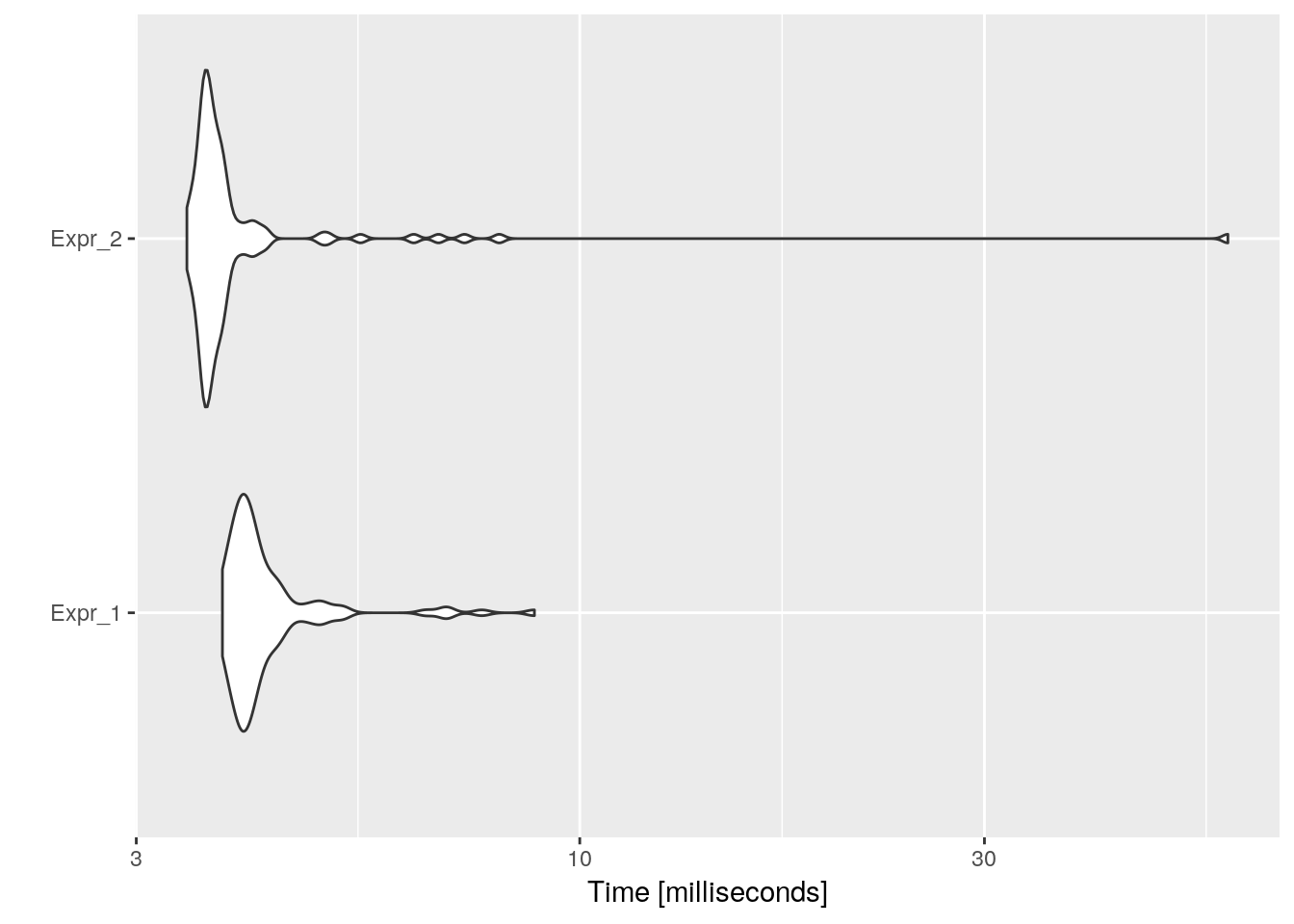``speed_up(mb_res)``
``````##            Min.  1st Qu.   Median      Mean  3rd Qu.      Max.
## Expr_2 1.101255 1.099989 1.110895 0.9748634 1.131798 0.1521909``````

#### Software pipelining

``# The loop is restructured in such a way that work done in an iteration is split into several parts and done over several iterations. In a tight loop this technique hides the latency between loading and using values.``

Comment: No example

#### Automatic parallelization

``# A loop is converted into multi-threaded or vectorized (or even both) code in order to utilize multiple processors simultaneously in a shared-memory multiprocessor (SMP) machine, including multi-core machines.``

Comment: No example

### Data-flow optimizations

``# Data-flow optimizations, based on data-flow analysis, primarily depend on how certain properties of data are propagated by control edges in the control flow graph. Some of these include:``

#### Common subexpression elimination

``# In the expression (a + b) - (a + b)/4, "common subexpression" refers to the duplicated (a + b). Compilers implementing this technique realize that (a + b) will not change, and so only calculate its value once.``
``````# 1
restore_sess()
b <- rnorm(1)
c <- rnorm(1)
g <- rnorm(1)
e <- rnorm(1)
mb_res <- microbenchmark(
{
a = b * c + g;
d = b * c * e;
f = b * c * 2;
g = (b * c) ^ 2;
},{
tmp = b * c;
a = tmp + g;
d = tmp * e;
f = tmp * 2;
g = (tmp) ^ 2;
}
)
autoplot(mb_res)``````
``## Coordinate system already present. Adding new coordinate system, which will replace the existing one.``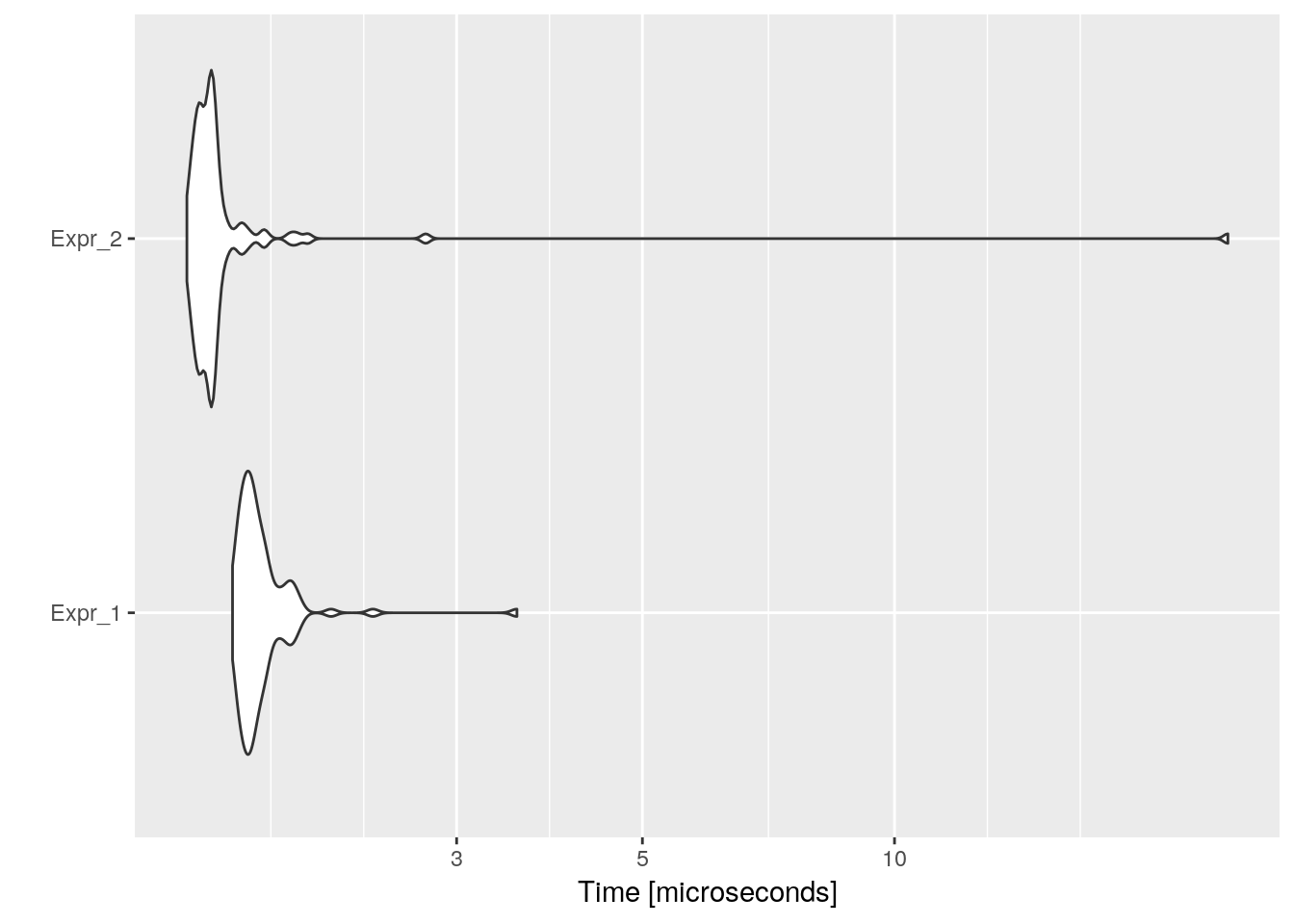``speed_up(mb_res)``
``````##           Min.  1st Qu.   Median     Mean  3rd Qu.     Max.
## Expr_2 1.13366 1.131294 1.124548 0.989587 1.148388 0.141589``````

#### Constant folding and propagation

``# replacing expressions consisting of constants (e.g., 3 + 5) with their final value (8) at compile time, rather than doing the calculation in run-time. Used in most modern languages.``
``````# constant folding
restore_sess()
mb_res <- microbenchmark(
{
i = 320 * 200 * 32;
},{
i = 2048000;
}
)
autoplot(mb_res)``````
``## Coordinate system already present. Adding new coordinate system, which will replace the existing one.``